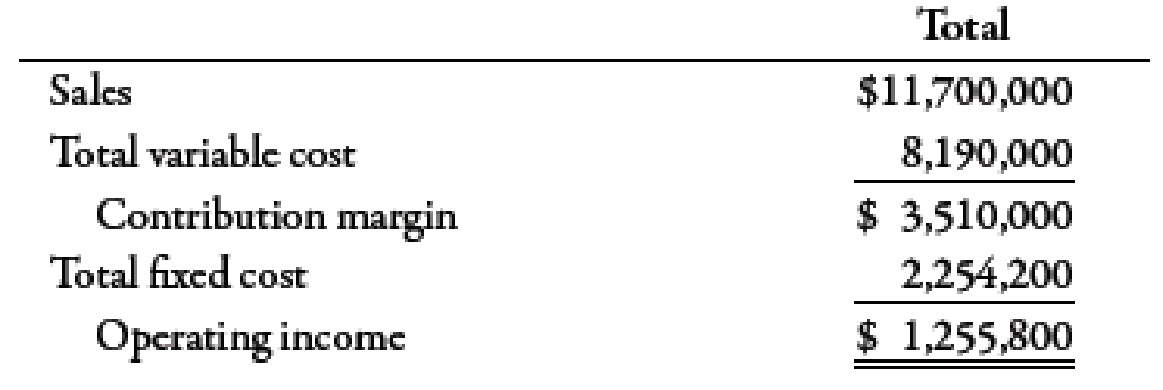Chapter 7, Problem 50E### Managerial Accounting: The Corners...

7th Edition
Maryanne M. Mowen + 2 others
ISBN: 9781337115773

#### Solutions

Chapter
Section### Managerial Accounting: The Corners...

7th Edition
Maryanne M. Mowen + 2 others
ISBN: 9781337115773
Textbook Problem
959 views

# Jellico Inc.’s projected operating income (based on sales of 450,000 units) for the coming year is as follows:Required: 1. Compute: (a) variable cost per unit, (b) contribution margin per unit, (c) contribution margin ratio, (d) break-even point in units, and (e) break-even point in sales dollars. 2. How many units must be sold to earn operating income of $296,400? 3. Compute the additional operating income that Jellico would earn if sales were$50,000 more than expected. 4. For the projected level of sales, compute the margin of safety in units, and then in sales dollars. 5. Compute the degree of operating leverage. (Note: Round answer to two decimal places.) 6. Compute the new operating income if sales are 10% higher than expected.

1.

To determine

Calculate variable cost per unit, contribution margin per unit, contribution margin ratio, break-even point in units, and break-even points in sales dollars.

Explanation

(a)

Variable Cost Per Unit:

Variable cost per unit can be calculated by dividing the total variable cost by the number of units or by adding all the variable costs per unit. Variable costs can be defined as the costs which increase with the increase in output and decrease with the decrease in output.

Use the following formula to calculate variable cost per unit:

Variablecostperunit=TotalvariablecostNumberofunitssold

Substitute $8,190,000 for total variable cost and 450,000 units for number of units sold in the above formula. Variablecostperunit=$8,190,000450,000=$18.2 Therefore, the variable cost per unit is$18.2.

(b)

Contribution margin:

Contribution margin can be defined as the amount obtained after deducting the variable expense from sales revenue. It means the amount of sales left after covering the variable expenses.

Use the following formula to calculate contribution margin per unit:

Contributionmarginperunit=ContributionmarginNumberofunitssold

Substitute $3,510,000 for contribution margin and 450,000 units for number of units sold in the above formula. Contributionmarginperunit=$3,510,000450,000=$7 2. To determine Compute the number of units to be sold to earn operating income of$296,400.

3.

To determine

Calculate the additional operating income if sales were \$50,000 more than expected.

4.

To determine

Calculate margin of safety in units and in sale dollars.

5.

To determine

Calculate the degree of operating leverage.

6.

To determine

Calculate the new operating income if sales were 10% higher than expected.

### Still sussing out bartleby?

Check out a sample textbook solution.

See a sample solution

#### The Solution to Your Study Problems

Bartleby provides explanations to thousands of textbook problems written by our experts, many with advanced degrees!

Get Started

#### Should an economic model describe reality exactly?

Principles of Microeconomics (MindTap Course List)

#### WACC Midwest Electric Company (MKC) uses only debt and common equity. It can borrow unlimited amounts at an int...

Fundamentals of Financial Management, Concise Edition (with Thomson ONE - Business School Edition, 1 term (6 months) Printed Access Card) (MindTap Course List)

#### Identify eight forms and sources of waste.

Cornerstones of Cost Management (Cornerstones Series)